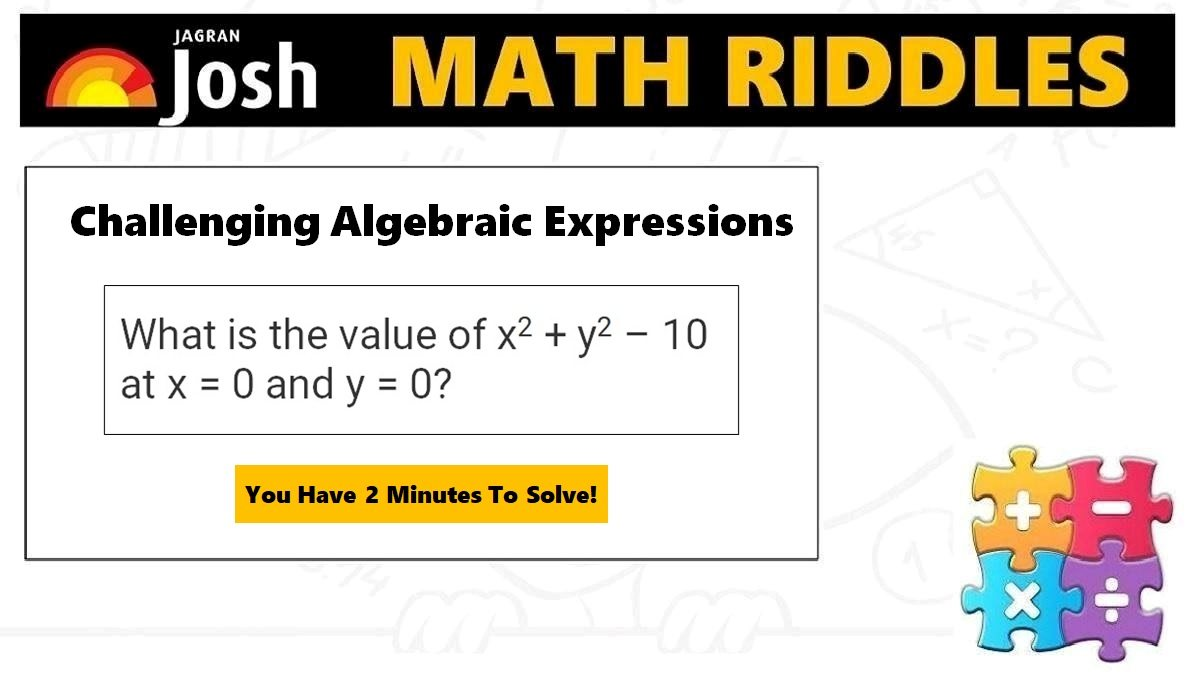# Math Riddles with Answers: 5 Challenging Puzzles for Class 8 Algebraic Expressions

Math Riddles for Class 8: Check out Algebraic Expressions Class 8 Puzzles for Preparing Important Topics for Class 8 Mathematics. Learn the tricks and solutions for algebraic problems.Math Riddles with Answers: 5 Challenging Puzzles for Class 8 Algebraic Expressions

Math Riddles: Today we bring fun and interactive Algebra math puzzles for students to prepare better for Class 8 Important Topic which is Algebraic Expressions and Identities. Students can learn and develop their solving skills with Jagran Josh’s Math Riddles with Answers series. We have also provided the NCERT solutions which contain algebraic expressions and identities class 8 worksheets with solutions for each puzzle below. Students can first take up the challenge to solve these mathematical problems in less 2 minutes each.

## Algebraic Expressions and Identities: What are Expressions?

Algebra is a branch of Mathematics that involves the use of letters to represent numbers (unknown quantities). Algebra allows us to write rules and formulas using variables, constants, etc which is a powerful method of determining unknowns. Algebra comes in handy in solving puzzles and logical reasoning questions, math riddles, and brain teasers in everyday life. Since the letters are representing unknown numbers, we can perform mathematical operations on these letters too which leads to the study of Algebraic Expressions and Identities.

Examples of Algebraic Expressions: x + 3, 4xy + 7, 2y – 5, etc

Algebraic Expressions are formed using variables (letters) and constants. In the expression 4xy + 7, the x and y are the variables whereas 7 is the constant. The value of the expression changes with the value chosen for the variables it contains. Thus, as y takes on different values, the value of 2y – 5 goes on changing. When y = 2, 2y – 5 = 2(2) – 5 = –1; when y = 0, 2y – 5 = 2 × 0 –5 = –5, etc.

### Terms, Factors, and Coefficients

Take the term 2y -5. This expression is made up of two terms, 2y and 5. Terms are added to form expressions. Terms themselves can be formed as the product of factors. The term 2y is the product of 2 and y. The term 5 is made up of just one factor, i.e., 5.

Another example, 3xy – 4x has two terms 3xy and 4x. The term 3xy is a product of factors 3, x, and y. The numerical factor of a term is called its numerical coefficient or simply coefficient. The coefficient in the term 3xy is 3 and the coefficient in the term 4x is 4.

### Monomials, Binomials, and Polynomials

Monomials: Expressions that contain only one term are called Monomials.

Binomials: Expressions that contain two terms are called Binomials.

Trinomials: Expressions containing three terms are called Trinomials.

Polynomials: Expressions containing, one or more terms with a non-zero coefficient (with variables having non-negative exponents) are called Polynomials. A polynomial may contain any number of terms, one or more than one.

## Algebraic Expressions and Identities: What are Identities?

We shall look into 3 identities that are useful to learn in Algebraic Expressions. These identities are obtained by multiplying a binomial by another binomial.

Let us solve a few challenging Algebraic Expressions and Identities questions.

Explanation:

Explanation:

Explanation:

Explanation:

Explanation:

## Tell us in comments: Did you solve these Algebraic Expressions questions in less than 2 minute each?

Check out more math puzzles!

Math Riddles with Answers: 95% People Could Not Solve This Math Problem, Can You?